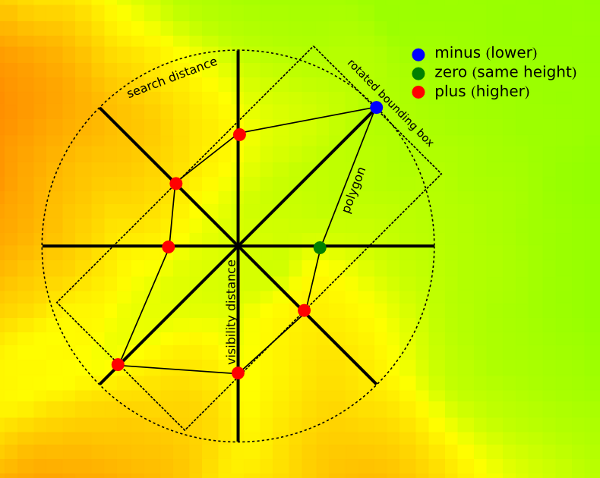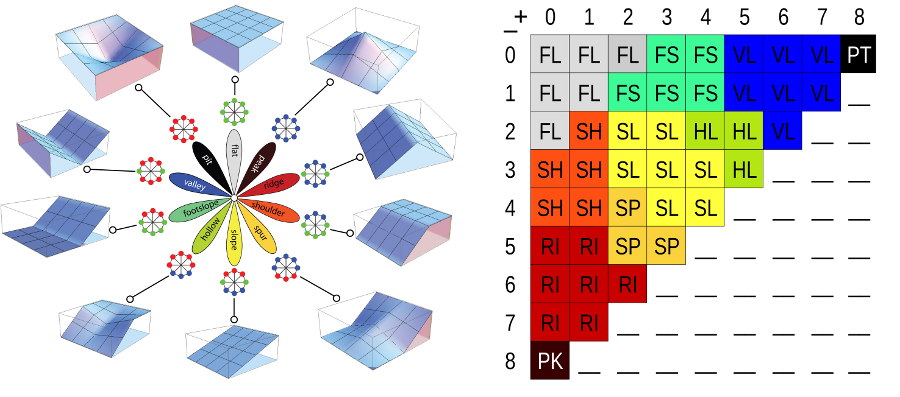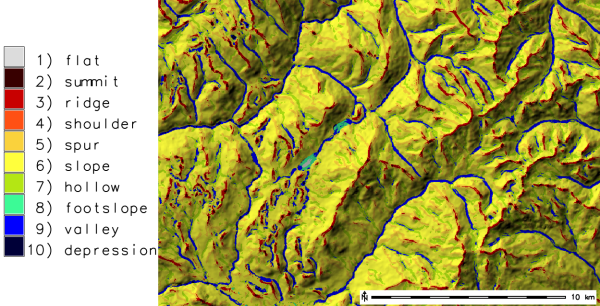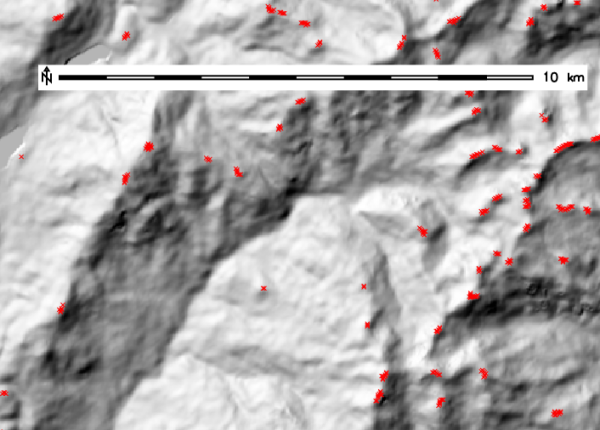## NAME

r.geomorphon - Calculates geomorphons (terrain forms) and associated geometry using machine vision approach.

## KEYWORDS

raster, geomorphons, terrain patterns, machine vision geomorphometry

## SYNOPSIS

r.geomorphon
r.geomorphon --help
r.geomorphon [-me] elevation=name [forms=name] [ternary=name] [positive=name] [negative=name] [intensity=name] [exposition=name] [range=name] [variance=name] [elongation=name] [azimuth=name] [extend=name] [width=name] search=integer skip=integer flat=float dist=float [prefix=string] [step=float] [start=float] [--overwrite] [--help] [--verbose] [--quiet] [--ui]

### Flags:

-m
Use meters to define search units (default is cells)
-e
Use extended form correction
--overwrite
Allow output files to overwrite existing files
--help
Print usage summary
--verbose
Verbose module output
--quiet
Quiet module output
--ui
Force launching GUI dialog

### Parameters:

elevation=name [required]
Name of input elevation raster map
forms=name
Most common geomorphic forms
ternary=name
Code of ternary patterns
positive=name
Code of binary positive patterns
negative=name
Code of binary negative patterns
intensity=name
Rasters containing mean relative elevation of the form
exposition=name
Rasters containing maximum difference between extend and central cell
range=name
Rasters containing difference between max and min elevation of the form extend
variance=name
Rasters containing variance of form boundary
elongation=name
Rasters containing local elongation
azimuth=name
Rasters containing local azimuth of the elongation
extend=name
Rasters containing local extend (area) of the form
width=name
Rasters containing local width of the form
search=integer [required]
Default: 3
skip=integer [required]
Default: 0
flat=float [required]
Flatenss treshold (degrees)
Default: 1
dist=float [required]
Flatenss distance, zero for none
Default: 0
prefix=string
Prefix for maps resulting from multiresolution approach
step=float
Distance step for every iteration (zero to omit)
Default: 0
start=float
Distance where serch will start in multiple mode (zero to omit)
Default: 0

## DESCRIPTION

### What is geomorphon:Geomorphon is a new concept of presentation and analysis of terrain forms. This concept utilises 8-tuple pattern of the visibility neighbourhood and breaks well known limitation of standard calculus approach where all terrain forms cannot be detected in a single window size. The pattern arises from a comparison of a focus pixel with its eight neighbors starting from the one located to the east and continuing counterclockwise producing ternary operator. For example, a tuple {+,-,-,-,0,+,+,+} describes one possible pattern of relative measures {higher, lower, lower, lower, equal, higher, higher, higher} for pixels surrounding the focus pixel. It is important to stress that the visibility neighbors are not necessarily an immediate neighbors of the focus pixel in the grid, but the pixels determined from the line-of-sight principle along the eight principal directions. This principle relates surface relief and horizontal distance by means of so-called zenith and nadir angles along the eight principal compass directions. The ternary operator converts the information contained in all the pairs of zenith and nadir angles into the ternary pattern (8-tuple). The result depends on the values of two parameters: search radius (L) and relief threshold (d). The search radius is the maximum allowable distance for calculation of zenith and nadir angles. The relief threshold is a minimum value of difference between LOSs angle (zenith and nadir) that is considered significantly different from the horizon. Two lines-of-sight are necessary due to zenith LOS only, does not detect positive forms correctly.

There are 38 = 6561 possible ternary patterns (8-tuplets). However by eliminating all patterns that are results of either rotation or reflection of other patterns wa set of 498 patterns remain referred as geomorphons. This is a comprehensive and exhaustive set of idealized landforms that are independent of the size, relief, and orientation of the actual landform.

Form recognition depends on two free parameters: Search radius and flatness threshold. Using larger values of L and is tantamount to terrain classification from a higher and wider perspective, whereas using smaller values of L and is tantamount to terrain classification from a local point of view. A character of the map depends on the value of L. Using small value of L results in the map that correctly identifies landforms if their size is smaller than L; landforms having larger sizes are broken down into components. Using larger values of L allows simultaneous identification of landforms on variety of sizes in expense of recognition smaller, second-order forms. There are two addational parameters: skip radius used to eliminate impact of small irregularities. On the contrary flatness distance eliminates the impact of very high distance (in meters) of search radius which may not detect elevation difference if this is at very far distance. Important especially with low resolution DEMS.

## OPTIONS

-m
All distance parameters (search, skip, flat distances) are supplied as meters instead of cells (default). To avoid situation when supplied distances is smaller than one cell program first check if supplied distance is longer than one cell in both NS and WE directions. For LatLong projection only NS distance checked, because in latitude angular unit comprise always bigger or equal distance than longitude one. If distance is supplied in cells, For all projections is recalculated into meters according formula: `number_of_cells*resolution_along_NS_direction`. It is important if geomorphons are calculate for large areas in LatLong projecton.
elevation
Digital elevation model. Data can be of any type and any projection. During calculation DEM is stored as floating point raster.
search
Determines length on the geodesic distances in all eight directions where line-of-sight is calculated. To speed up calculation is determines only these cells which centers falls into the distance
skip
Determines length on the geodesic distances at the beginning of calculation all eight directions where line-of-sight is yet calculated. To speed up calculation this distance is always recalculated into number of cell which are skipped at the begining of every line-of-sight and is equal in all direction. This parameter eliminates forms of very small extend, smaller than skip parameter.
flat
The difference (in degrees) between zenith and nadir line-of-sight which indicate flat direction. If higher threshold produce more flat maps. If resolution of the map is low (more than 1 km per cell) threshold should be very small (much smaller than 1 degree) because on such distance 1 degree of difference means several meters of high difference.
dist
>Flat distance. This is additional parameter defining the distance above which the threshold starts to decrease to avoid problems with pseudo-flat line-of-sights if real elevation difference appears on the distance where its value is higher DO POPRAWKI
form
Returns geomorphic map with 10 most popular terrestial forms. Legend for forms, its definition by the number of + and - and its idealized visualisation are presented at the image.

### Forms represented by geomorphons:pattern
returns code of one of 498 unique ternary patterns for every cell. The code is a decimal representation o 8-tuple minimalised patterns written in ternary system. Full list of patterns is available in source code directory as patterns.txt. This map can be used to create alternative form classification using supervised approach
positive and negative
returns codes binary patterns for zenith (positive) and nadir (negative) line of sights. The code is a decimal representation o 8-tuple minimalised patterns written in binary system. Full list of patterns is available in source code directory as patterns.txt

NOTE: parameters below are very experimental. The usefulness of these parameters are currently under investigation

intensity
returns avarage difference between central cell of geomorphon and eight cells in visibility neighbourhood. This parameter shows local (as is visible) exposition/abasment of the form in the terrain
range
returns difference between minimum and maximum values of visibility neigbourhood.
variance
returns variance (difference between particular values and mean value) ofvisibility neigbourhood.
extend
returns area of the polygon created by the 8 points where line-of-sight cuts the terrain (see image in description section).
azimuth
returns orientation of the poligon constituting geomorphon. This orientation is currentlyb calculated as a orientation of least square fit line to the eight verticles of this polygon.
elongation
returns proportion between sides of the bounding box rectangle calculated for geomorphon rotated to fit lest square line.
width
returns lenght of the shorter side of the bounding box rectangle calculated for geomorphon rotated to fit lest square line.

## NOTES

From computational point of view there are no limitations of input DEM and free parameters used in calculation. However, in practice there are some issues on DEM resolution and search radius. Low resolution DEM especially above 1 km per cell requires smaller than default flatness threshold. On the other hand, only forms with high local elevation difference will be detected correctly. It results form fact that on very high distance (of order of kilometers or higher) even relatively high elevation difference will be recognized as flat. For example at the distance of 8 km (8 cells with 1 km resolution DEM) an relative elevation difference of at least 136 m is required to be noticed as non-flat. Flatness distance threshold may be helpful to avoid this problem.

## EXAMPLES

### Geomorphon calculation: extraction of terrestial landforms

Geomorphon calculation example using the EU DEM 25m:
```g.region raster=eu_dem_25m -p
r.geomorphon elevation=eu_dem_25m forms=eu_dem_25m_geomorph

# verify terrestial landforms found in DEM
r.category eu_dem_25m_geomorph
1  flat
2  summit
3  ridge
4  shoulder
5  spur
6  slope
7  hollow
8  footslope
9  valley
10 depression
```### Extraction of summits

Using the resulting terrestial landforms map, single landforms can be extracted, e.g. the summits, and converted into a vector point map:
```r.mapcalc expression="eu_dem_25m_summits = if(eu_dem_25m_geomorph == 2, 1, null())"
r.thin input=eu_dem_25m_summits output=eu_dem_25m_summits_thinned
r.to.vect input=eu_dem_25m_summits_thinned output=eu_dem_25m_summits type=point
v.info input=eu_dem_25m_summits
```r.param.scale

## REFERENCES

• Stepinski, T., Jasiewicz, J., 2011, Geomorphons - a new approach to classification of landform, in : Eds: Hengl, T., Evans, I.S., Wilson, J.P., and Gould, M., Proceedings of Geomorphometry 2011, Redlands, 109-112 (PDF)
• Jasiewicz, J., Stepinski, T., 2013, Geomorphons - a pattern recognition approach to classification and mapping of landforms, Geomorphology, vol. 182, 147-156 (DOI: 10.1016/j.geomorph.2012.11.005)

## AUTHORS

Jarek Jasiewicz, Tomek Stepinski (merit contribution)

## SOURCE CODE

Available at: r.geomorphon source code (history)

© 2003-2020 GRASS Development Team, GRASS GIS 7.8.3dev Reference Manual